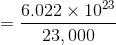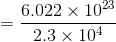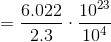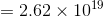# PSAT Math : How to divide decimals

## Example Questions

### Example Question #7 : Decimal Operations

simplify: 0.2 ÷ 0.04

0.05
5
0.02
0.005
2
Explanation:

0.2 ÷ 0.04  <- easiest way to approach is to move the decimal to the right (the same amount of spaces on each side) so that the decimal disappears

since 0.04 has 2 spaces and 0.2 only has 1, move the decimal 2 spaces to the right on each side

0.2 ÷ 0.04 = 20 ÷ 4 = 5

### Example Question #8 : Decimal Operations

If the cost of 20 equally priced pencils cost $5.80, then at the same rate what is the greatest number of pencils you can purchase for$50?

200

172.41

150

173

172

172

Explanation:

Remeber for this problem that you cannot spend more than $50. First find the price of each pencil by 5.80/20 = 29 cents. And then use this to do 50/0.29 = 172.41. So the greatest number of pencils you can purchase for$50 is 172 pencils.

### Example Question #1 : How To Divide Decimals

Charlie has charges on his credit card of $63.70,$37.32, and $81.78. If he pays down half his bill at once, and then pays the rest in four equal monthly installments, how much is each monthly installment? Possible Answers: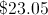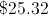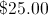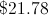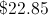Correct answer:Explanation: Adding all the charges together and dividing by 2 yields$91.40. Dividing this by 4 yields a monthly installment of \$22.85.

### Example Question #1 : How To Divide Decimals

Simplify: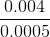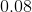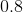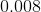Explanation:

Multiply both the numerator and denominator by some power of 10 to get rid of the decimals; in this case, multiply both by 10,000.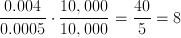### Example Question #11 : Decimal Operations

Which number has 2.8 as its reciprocal?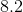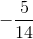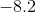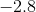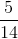Explanation:

The number that has 2.8 as its reciprocal is, in return, the reciprocal of 2.8.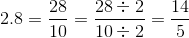The reciprocal of this can be found by switching the numerator and the denominator. This is.

### Example Question #502 : Arithmetic

23 grams of sodium are equal to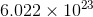atoms. How many atoms comprise one milligram of sodium?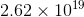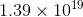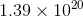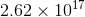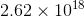Explanation:

23 grams are equal to 23,000 milligrams, so to find the number of atoms in one milligram, divide: